# HIDIHO!

### giving something back to the Flash community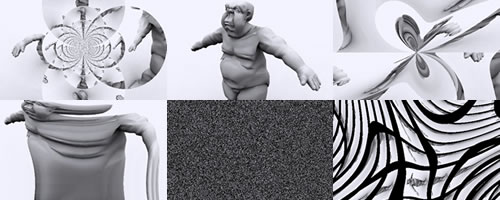to make shader, we start by normalizing the space (at least in french and if I got it right) ; a coordinates system is normalized when all the image’s coordinates are bound between -1 & 1 on x & y
might sound stupid but it’s not.
it is also something we do for the strange attractors, to know their size and be able to plot them.
So first we have to normalize the space, here’s the basis I use:

```<languageversion : 1.0;>
kernel Distort
< namespace : "net.nicoptere.filters";
version : 1;
description : "normalized coordinates";
>
{
input image4 src;
output pixel4 dst;

parameter float2 imageSize
< minValue:float2( 2.0, 2.0 );
maxValue:float2( 256.0, 256.0 );
defaultValue:float2( 256.0, 256.0 );
>;
parameter float2 center
< minValue:float2( 0.0, 0.0 );
maxValue:float2( 256.0, 256.0 );
defaultValue:float2( 128.0, 128.0 );
>;
void
evaluatePixel()
{
//offsets the image
float2 pos = outCoord() - center;

//creates a normalized coordinates system
float W = imageSize.x;
float H = imageSize.y;

float x = pos.x;
float y = pos.y;

float u = x / W;
float v = y / H;
float w = 0.0;

//a couple of useful variables
float pi = 3.14159265358979;
float radian = ( pi / 180.0 );
float r = sqrt( u*u + v*v );
float a = atan( v, u );

//tweak here

u = cos( a ) * dot( u,u );
v = sin( a ) * dot( v,v );

//stop tweaking

//feedback loop
if( u > 1.0 )   u -= floor( u );
if( v > 1.0 )   v -= floor( v );
if( u < -1.0 )  u += ceil( -u );
if( v < -1.0 )  v += ceil( -v );

//rescale
u *= W;
v *= H;

dst = sampleNearest(src,float2(center.x+u, center.y+v ));
}
}```

so:
we start by shifting the current pixel:

```    	//offsets the image
float2 pos = outCoord() - center;
```

then we divide it by the total size.

```        //creates a normalized coordinates system
float u = pos.x / imageSize.x;
float v = pos.y / imageSize.y;```

then we tweak the U/V (+eventuellay W) that’s where you can do lots of things. the values in this case are:

```	u = cos( a ) * dot( u,u );
v = sin( a ) * dot( v,v );```

then we add create a feedback loop so that pixel remain in the window: it increases the complexity

```	//feedback loop
if( u > 1.0 )   u -= floor( u );
if( v > 1.0 )   v -= floor( v );
if( u < -1.0 )  u += ceil( -u );
if( v < -1.0 )  v += ceil( -v );
```

after which we rescale the coordinates backwards
[/actionscript3]

```	//rescale
u *= imageSize.x;
v *= imageSize.y;```

and finally we shift them again

`	dst = sampleNearest(src,float2(center.x+u, center.y+v ));`

that’s it.
here’s an example of the code above:

so if you have understood the explainations above, you can try the code below in PB.
remember to change the width/height of the input picture ; it gives pretty interesting results.

starting with the image hereunder: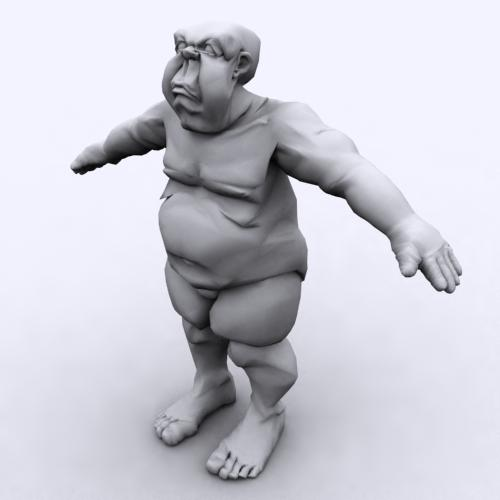and this equation:

```	u = cos( a ) * dot( u,u );
v = sin( a ) * dot( v,v );```

you hould get :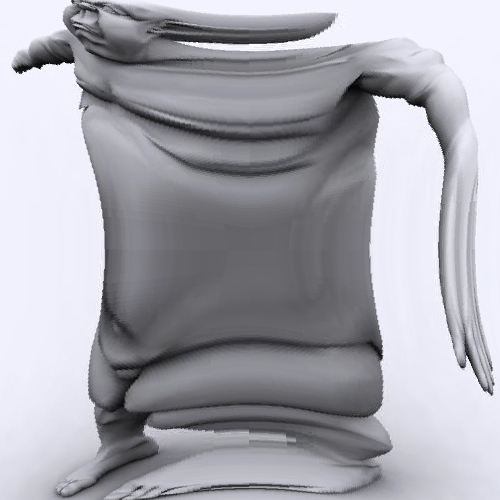with

```	u = cos( a ) / r;
v = sin( a ) / r;```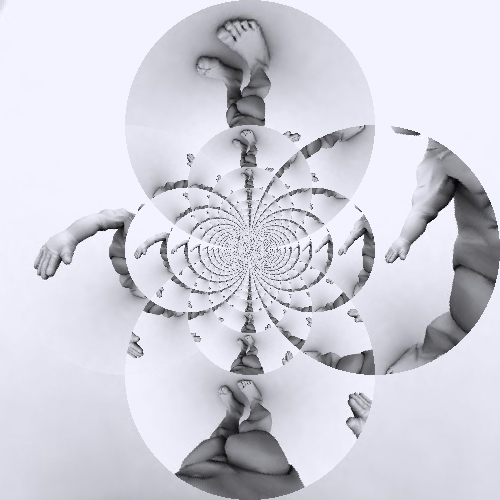I noticed that there’s no Random() method in PixelBender which is a shame. after tweaking a bit I found a way to get a decently random noise:

```    float mess = x * H + y;
u = cos( mess / 180.0 * pi ) * 1.0/mess;
v = sin( mess / 180.0 * pi ) * mess / r;```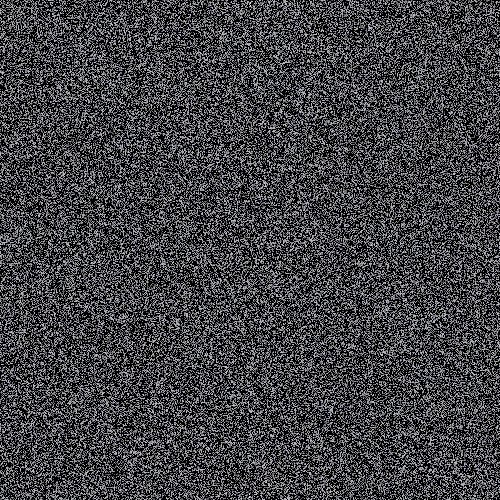there’s also that website mathCurve where lots of 2D functions are to be found and adaptd for instance the quintic curve:

```    //http://www.mathcurve.com/courbes2d/quintic/quintic.shtml
u += ( r * sin( a ) ) / 1.0 + cos( a ) * cos( 2.0 * a );
v += ( r * sin( a ) ) / 1.0 + cos( a ) * cos( 2.0 * a );```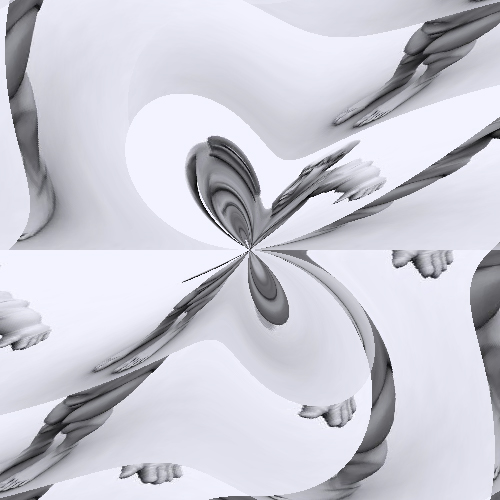or the regular bifolium:

```    //http://www.mathcurve.com/courbes2d/bifoliumregulier/bifoliumregulier.shtml
u += 4.0 * r * sin( a ) * dot( cos( a ), cos( a ) );
v += 4.0 * r * sin( a ) * dot( cos( a ), cos( a ) );
```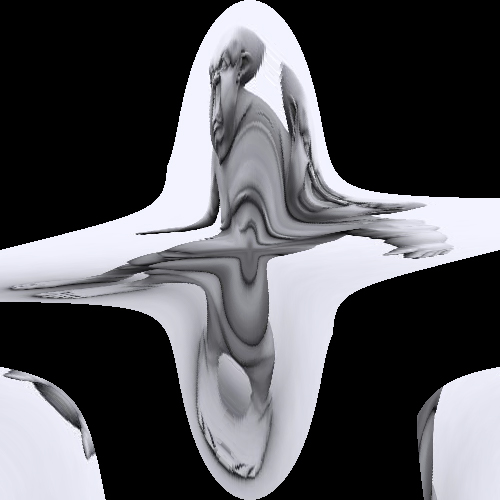btw I stumbled upon two excellent resources:

at iñigo’s, there are very neat distorsions for instance (it’s still the same base image)

```    u = x * cos( 2.0 * r ) - y * sin( 2.0 * r );
v = y * cos( 2.0 * r ) + x * sin( 2.0 * r );
``````    r *= 2.0;
u = 0.02 * y + cos( a*3.0)*r*r;
v = 0.02 * x + sin( a*3.0)*r*r;
```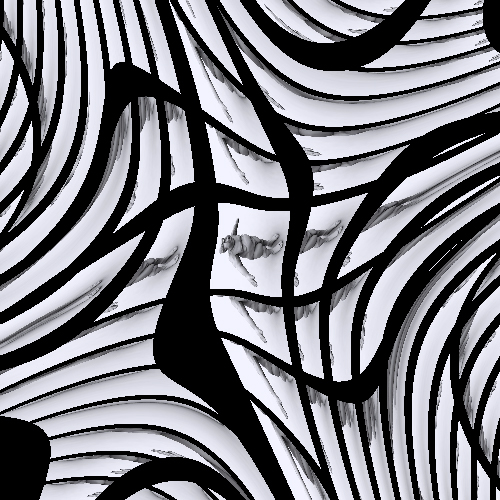another great resources is Paul Bourke’s geometry webpages:
for instance the epicycloid is written:

```        u += (r2 + r1) * cos(a) - r1 * cos( (r2 + r1) * a / r1);
v += (r2 + r1) * sin(a) - r1 * sin( (r2 + r1) * a / r1);
```

with r1 = -.1 and r2 = -.3 will give us: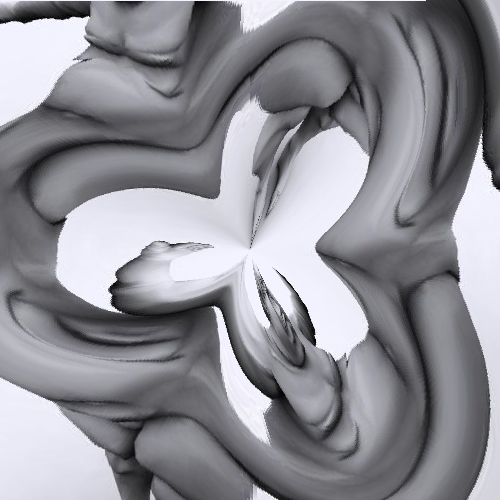the folium :

```        r = 3.0 * cos(t) * sin(t) / (cos(t) * 3.0 + sin(t) * 3.0 ) ;
u = cos( a ) * r;
v = sin( a ) * r;```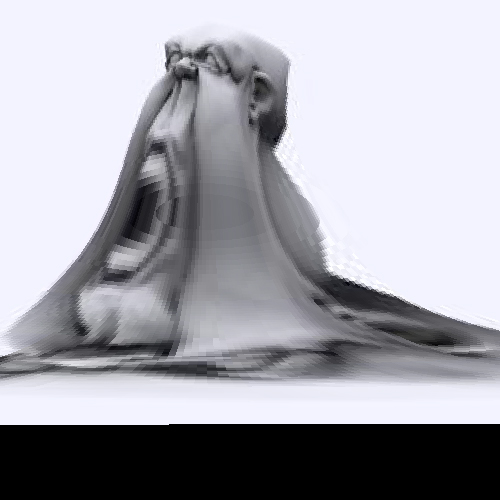this is quite endless and I spent a couple of days tweaking, testing blahblah.
next posts should talk about projection but not sure. maybe I’ll post a ‘tips and tricks’ article if I gather enough interesting things.

to be followed…